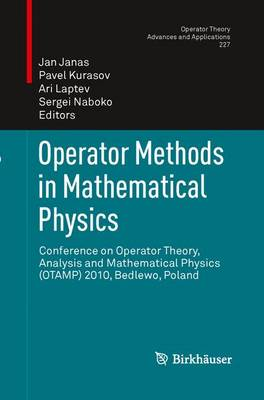•# Operator Methods in Mathematical Physics: Conference on Operator Theory, Analysis and Mathematical Physics (OTAMP) 2010, Bedlewo, Poland - Operator Theory: Advances and Applications 227 (Paperback)

(editor), (editor), (editor), (editor)
£90.00
Paperback 178 Pages / Published: 20/06/2015
• We can order this from the publisher

Usually dispatched within 15 working days

The conference Operator Theory, Analysis and Mathematical Physics - OTAMP is a regular biennial event devoted to mathematical problems on the border between analysis and mathematical physics. The current volume presents articles written by participants, mostly invited speakers, and is devoted to problems at the forefront of modern mathematical physics such as spectral properties of CMV matrices and inverse problems for the non-classical Schroedinger equation. Other contributions deal with equations from mathematical physics and study their properties using methods of spectral analysis. The volume explores several new directions of research and may serve as a source of new ideas and problems for all scientists interested in modern mathematical physics.

Publisher: Birkhauser Verlag AG
ISBN: 9783034807876
Number of pages: 178
Weight: 2934 g
Dimensions: 235 x 155 x 10 mm
Edition: 2013 ed.